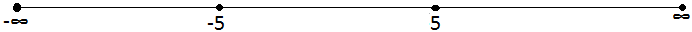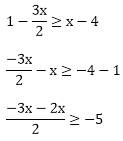×#### Thank you for registering.

One of our academic counsellors will contact you within 1 working day.

Click to Chat

1800-1023-196

+91-120-4616500

CART 0

• 0

MY CART (5)

Use Coupon: CART20 and get 20% off on all online Study Material

ITEM
DETAILS
MRP
DISCOUNT
FINAL PRICE
Total Price: Rs.

There are no items in this cart.
Continue Shopping• Complete JEE Main/Advanced Course and Test Series
• OFFERED PRICE: Rs. 15,900
• View Details

```Chapter 15: Linear Inequations – Exercise 15.2

Linear Inequations – Exercise – 15.2 – Q.1

Consider the first inequation,

x + 3 > 0

x > - 3         .... (i)

Consider the second inequation,

2x < 14

x < 14/2 = 7

x < 7         …. (ii)

From (i) and (ii), (- 3, 7) is the solution set of the simultaneous equations.

Linear Inequations – Exercise – 15.2 – Q.2

Consider the first inequation,

2x – 7 > 5 - x

⟹ 2x + x > 5 + 7

⟹  3x > 12

⟹ x >12/3

⟹ x > 4          …….. (i)

Consider the second inequation,

11 - 5x ≤ 1

⟹ - 5x ≤ 1 - 11

⟹ - 5x ≤ - 10

⟹ 5x ≥ 10

⟹ x ≥ 2       …….. (ii)

From (i) and (ii), (4, ∞) is the solution set of the simultaneous equtions.

Linear Inequations – Exercise – 15.2 – Q.3

Consider the first inequation,

x - 2 > 0

x > 2        .... (i)

Consider the second inequation,

3x < 18

x < 6       ....... (ii)

From (i) and (ii), (2, 6) is the solution set of the simultaneous equations.

Linear Inequations – Exercise – 15.2 – Q.4

Consider the first inequation,

2x + 6 ≥ 0

2x ≥ - 6

x ≥ (-6)/2

x ≥ - 3            …….. (i)

consider the second inequation,

4x – 7 < 0

4x < 7

x < 7/4            ……. (ii)

From (i) and (ii), [- 3, 7/4] is the solution set of the simultaneous equations.

Linear Inequations – Exercise – 15.2 – Q.5

Consider the first inequation,

3x – 6 > 0

3x > 6

x > 2          …….. (i)

Consider the second inequation,

2x – 5 > 0

2x > 5

x > 5/2            …. (ii)

From (i) and (ii), [5/2, ∞] is the solution set of the simultaneous equations.

Linear Inequations – Exercise – 15.2 – Q.6

Consider the first inequation,

2x – 3 < 7

2x < 7 + 3

2x < 10

x < 5                ….. (i)

Consider the second inequation,

2x > - 4

x > (-4)/2

x > - 2           ….. (ii)

From (i) and (ii), [-2, 5] is the solution set of the simultaneous equations.

Linear Inequations – Exercise – 15.2 – Q.7

Consider the first inequation,

2x + 5 ≤ 0

2x ≤ - 5

x ≤ (-5)/2       …… (i)

Consider the second inequation,

x – 3 ≤ 0

x ≤ 3         …… (ii)

From (i) and (ii), (-∞, – 5/2] is the solution set of the simultaneous equations.

Linear Inequations – Exercise – 15.2 – Q.8

5x – 1 < 24

5x < 24 + 1

5x < 25

x < 25/5

x < 5      …… (1)

and

5x + 1 > - 24

5x > - 24 - 1

5x > - 25

x > - 5          .…. (2)

From equation (1) and (2),

< x < 5

⟹ (- 5, 5)Linear Inequations – Exercise – 15.2 – Q.9

Consider the first inequation,

3x - 1 ≥ 5

3x ≥ 5 + 1

3x ≥ 6

x ≥ 2   ..... (i)

Consider the second inequatin,

x + 2 > -1

x > -1 -2

x > -3            ...... (ii)

From (i) and (ii), [2, ∞] is the solution set of the simultaneous equations.

Linear Inequations – Exercise – 15.2 – Q.10

Consider the first inequation,

11 - 5x > -4

-5x > -4 - 11

-5x < -15

5x < 15

x < 3 .... (i)

Consider the second inequation,

4x + 13 ≤ -11

4x ≤ -11 - 13

4x ≤ -24

x ≤ -6 ...(ii)

From (i) and (ii), [-∞,-6] is the solution set of the simultaneous equations.

Linear Inequations – Exercise – 15.2 – Q.11

Consider the first inequation,

4x – 1 ≤ 0

4x > - 1

- 5x ≤ - 15

x ≤ 1/4          …..  (i)

Consider the second inequation,

3 - 4x < 0

- 4x < - 3

- x <- 3/4

x > 3/4           …… (ii)

From (i) and (ii), there is no solution set of the simultaneous equations.

Linear Inequations – Exercise – 15.2 – Q.12

Consider the first inequation,

x + 5 > 2(x + 1)

x > 2x + 2 - 5

x > 2x - 3

x - 2 > - 3

-x > - 3

x < 3       ......  (i)

Consider the second inequation,

2 - x < 3(x + 2)

2 - x < 3x + 6

-x - 3x < 6 - 2

- 4x < 4

x > -1     ..... (ii)

From (i) and (ii), (-1, 3) is the solution set of the simultaneous equations.

Linear Inequations – Exercise – 15.2 – Q.13

Consider the first inequation,

2(x - 6) < 3x - 7

⟹ 2x - 12 < 3x - 7

⟹ - 5 < x        ...... (i)

Consider the second inequation,

11 - 2x < 6 - x

-2x + x < 6 - 11

- x < - 5

x > 5           ....... (ii)

From (i) and (ii), (5, ∞) is the solution set of the simultaneous equations.

Linear Inequations – Exercise – 15.2 – Q.14

Consider the first inequation,

5x – 7 < 3(x + 3)

5x - 7< 3x + 9

5x - 3x < 9 + 7

2x < 16

x < 8             ……. (i)

Consider the second inequation,From (i) and (ii), (-∞, 2) is the solution set of the simultaneous equations.

```### Course Features

• 728 Video Lectures
• Revision Notes
• Previous Year Papers
• Mind Map
• Study Planner
• NCERT Solutions
• Discussion Forum
• Test paper with Video Solution